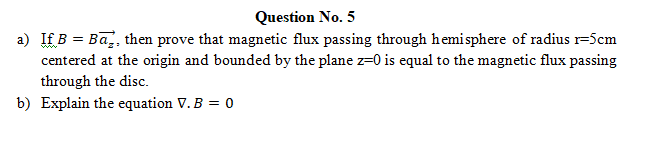# (Solved): Subject: Electromagnetic Field Theory please mention each step involving in the solution. Questi ...Subject: Electromagnetic Field Theory

please mention each step involving in the solution.

Question No. 5 a) If $$B=B \overrightarrow{a_{z}}$$, then prove that magnetic flux passing through hemisphere of radius $$\mathrm{r}=5 \mathrm{~cm}$$ centered at the origin and bounded by the plane $$z=0$$ is equal to the magnetic flux passing through the disc. b) Explain the equation $$\nabla \cdot B=0$$

We have an Answer from Expert